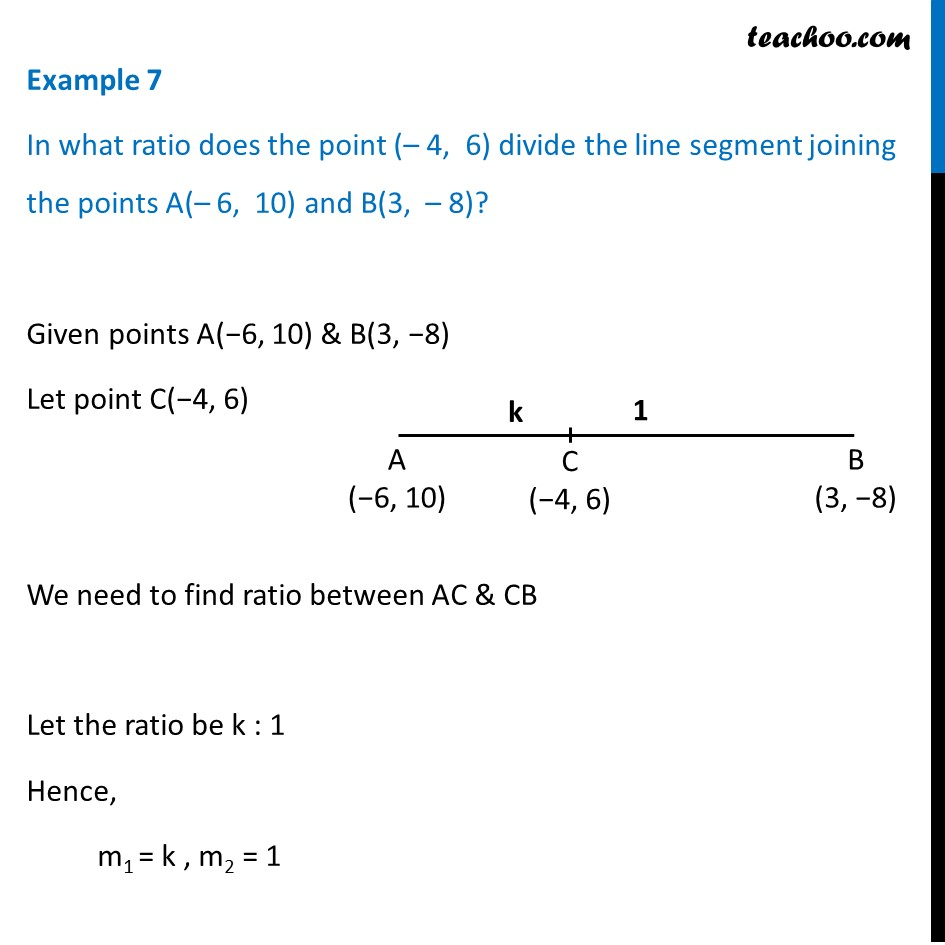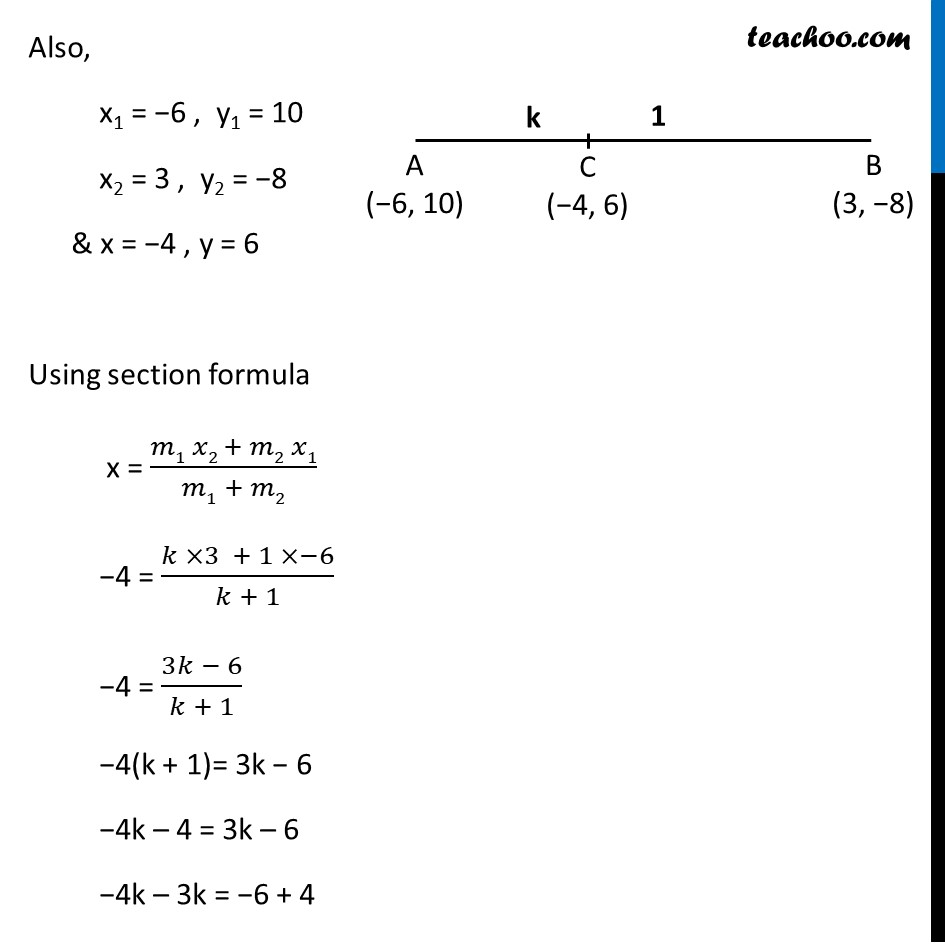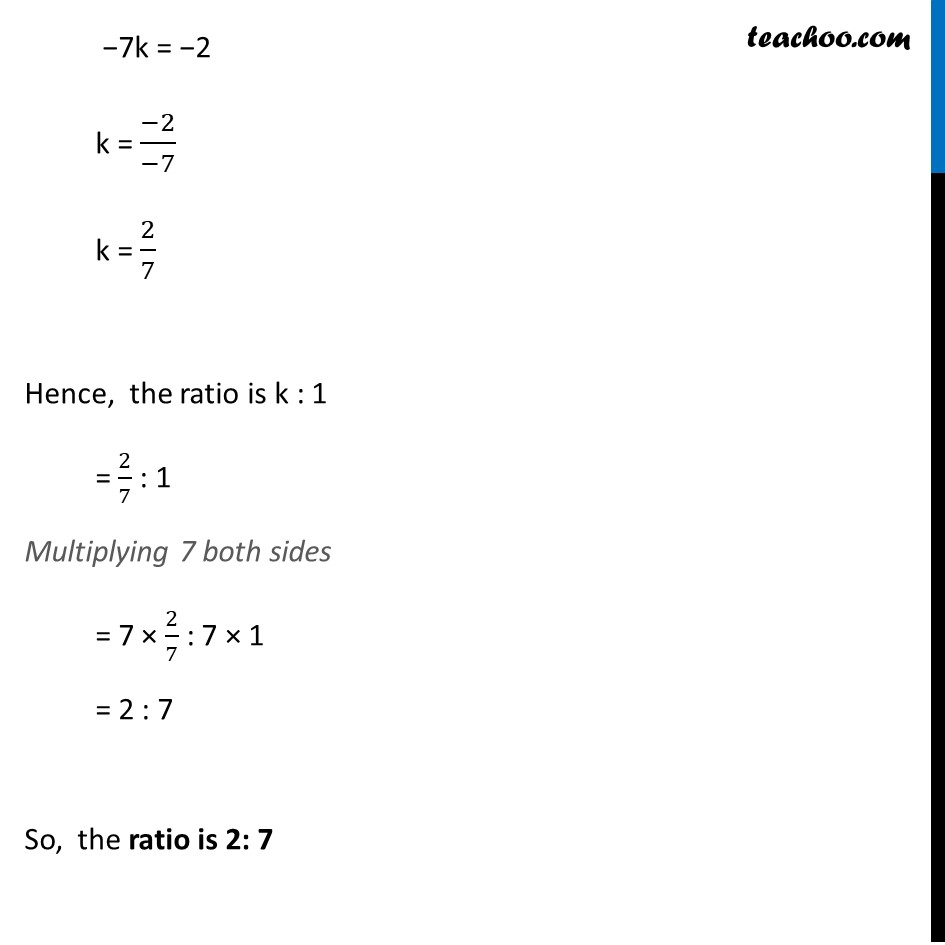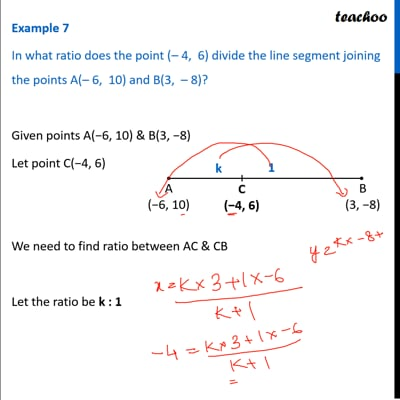Examples

Chapter 7 Class 10 Coordinate Geometry
Serial order wiseThis video is only available for Teachoo black users

### Transcript

Example 7 In what ratio does the point (– 4, 6) divide the line segment joining the points A(– 6, 10) and B(3, – 8)? Given points A(−6, 10) & B(3, −8) Let point C(−4, 6) We need to find ratio between AC & CB Let the ratio be k : 1 Hence, m1 = k , m2 = 1 Also, x1 = −6 , y1 = 10 x2 = 3 , y2 = −8 & x = −4 , y = 6 Using section formula x = (𝑚1 𝑥2 + 𝑚2 𝑥1)/(𝑚1 + 𝑚2) −4 = (𝑘 ×3 + 1 ×−6)/(𝑘 + 1) −4 = (3𝑘 − 6)/(𝑘 + 1) −4(k + 1)= 3k − 6 −4k – 4 = 3k – 6 −4k – 3k = −6 + 4 −7k = −2 k = (−2)/(−7) k = 2/7 Hence, the ratio is k : 1 = 2/7 : 1 Multiplying 7 both sides = 7 × 2/7 : 7 × 1 = 2 : 7 So, the ratio is 2: 7